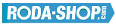# Stainless Steel Ball BearingsStainless Steel AISI 440C(Nr.1.4125)

Manufacturer Product Image Item Name Price### S61700

Thin Section Bearings Stainless Steel Open(No Shields) Dimension d=10.00 Dimension D=15.00 Dimension B=3.00 Dinamic Load Rating=855 Static Load Rating=435 Weight=0.002gr. Speed limit Grease=15.0 Speed limit Oil=17.0
inside diameter = 10.00
outside diameter = 15.00
width = 3.00
€13.31### S61700-2RS

Thin Section Bearings Stainless Steel Rubber Seals on Both sides Dimension d=10.00 Dimension D=15.00 Dimension B=4.00 Dinamic Load Rating=855 Static Load Rating=435 Weight=0.002gr. Speed limit Grease=15.0 Speed limit Oil=17.0
inside diameter = 10.00
outside diameter = 15.00
width = 4.00
€13.31### S61700-2Z

Thin Section Bearings Stainless Steel Metallic Shields on both sides Dimension d=10.00 Dimension D=15.00 Dimension B=4.00 Dinamic Load Rating=855 Static Load Rating=435 Weight=0.002gr. Speed limit Grease=15.0 Speed limit Oil=17.0
inside diameter = 10.00
outside diameter = 15.00
width = 4.00
€13.31### S61701

Thin Section Bearings Stainless Steel Open(No Shields) Dimension d=12.00 Dimension D=18.00 Dimension B=4.00 Dinamic Load Rating=926 Static Load Rating=530 Weight=0.004gr. Speed limit Grease=13.0 Speed limit Oil=15.0
inside diameter = 12.00
outside diameter = 18.00
width = 4.00
€13.92### S61701-2RS

Thin Section Bearings Stainless Steel Rubber Seals on Both sides Dimension d=12.00 Dimension D=18.00 Dimension B=4.00 Dinamic Load Rating=926 Static Load Rating=530 Weight=0.004gr. Speed limit Grease=13.0 Speed limit Oil=15.0
inside diameter = 12.00
outside diameter = 18.00
width = 4.00
€13.92### S61701-2Z

Thin Section Bearings Stainless Steel Metallic Shields on both sides Dimension d=12.00 Dimension D=18.00 Dimension B=4.00 Dinamic Load Rating=926 Static Load Rating=530 Weight=0.004gr. Speed limit Grease=13.0 Speed limit Oil=15.0
inside diameter = 12.00
outside diameter = 18.00
width = 4.00
€13.92### S61702

Thin Section Bearings Stainless Steel Open(No Shields) Dimension d=15.00 Dimension D=21.00 Dimension B=4.00 Dinamic Load Rating=937 Static Load Rating=582 Weight=0.004gr. Speed limit Grease=11.0 Speed limit Oil=13.0
inside diameter = 15.00
outside diameter = 21.00
width = 4.00
€14.52### S61702-2RS

Thin Section Bearings Stainless Steel Rubber Seals on Both sides Dimension d=15.00 Dimension D=21.00 Dimension B=4.00 Dinamic Load Rating=937 Static Load Rating=582 Weight=0.004gr. Speed limit Grease=11.0 Speed limit Oil=13.0
inside diameter = 15.00
outside diameter = 21.00
width = 4.00
€14.52### S61702-2Z

Thin Section Bearings Stainless Steel Metallic Shields on both sides Dimension d=15.00 Dimension D=21.00 Dimension B=4.00 Dinamic Load Rating=937 Static Load Rating=582 Weight=0.004gr. Speed limit Grease=11.0 Speed limit Oil=13.0
inside diameter = 15.00
outside diameter = 21.00
width = 4.00
€14.52### S61703

Thin Section Bearings Stainless Steel Open(No Shields) Dimension d=17.00 Dimension D=23.00 Dimension B=4.00 Dinamic Load Rating=1000 Static Load Rating=658 Weight=0.005gr. Speed limit Grease=9.5 Speed limit Oil=11.0
inside diameter = 17.00
outside diameter = 23.00
width = 4.00
€14.64